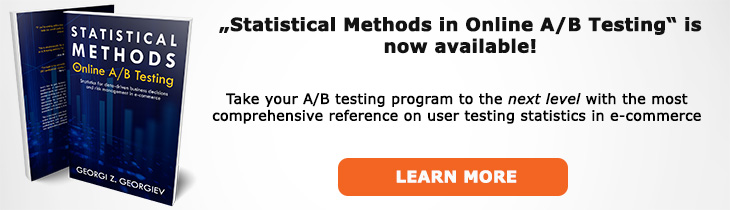# What does "Significance Level" mean?

Definition of Significance Level in the context of A/B testing (online controlled experiments).

## What is a Significance Level?

Aliases: observed significance

The term significance level is often used in A/B testing to denote the observed statistical significance (post-hoc) and it is usually communicated not as the p-value (as is common in the sciences) but as the confidence level: the inverse of the p-value (1 - p) as percentage which is mathematically valid due to the duality between p-values and confidence intervals. However, it can be terminologically confusing since the leap from saying the significance level of a test is 99% to saying "there is only 1% probability that the null hypothesis is false" - logically it does not follow.

More formally the significance level is the probability of a worse fit with the data at hand (x0): p(x0) = P(d(X) > d(x0); H0) which is exactly the definition of the p-value.

When properly understood, it is the confidence level of a confidence interval that would just barely cover the upper (or lower) limit of the null hypothesis. For example, if an A/B test is significant at the 99% level with a null hypothesis of δ ≤ 0 then the lower confidence limit of a 99% one-sided confidence interval will not cover 0.

## Related A/B Testing terms

Statistical Significance

alpha

Confidence Level

## Articles on Significance Level

Like this glossary entry? For an in-depth and comprehensive reading on A/B testing stats, check out the book "Statistical Methods in Online A/B Testing" by the author of this glossary, Georgi Georgiev.## Glossary Index by Letter

Select a letter to see all A/B testing terms starting with that letter or visit the Glossary homepage to see all.